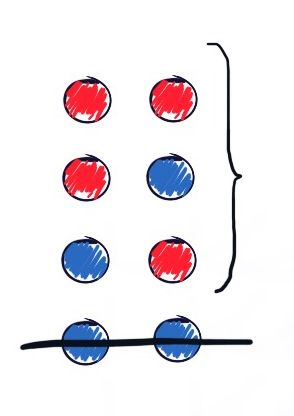This is the solution of Problem #1.4 from Probability Interview Questions.

### Problem statement

There are two balls in a bag. I tell you that at least one of them is red. What is the probability that both are red?

This is a simple counting problem. We're given 2 balls, each can be either red or not red.

With two balls like this, the set of all possible outcomes contains 4 outcomes that look like this:Sample space - all possible outcomes with two balls which are either Red or Not Red

Since we're told that at least one of the balls is red, our problem deals with a conditional universe:Conditional sample space (or conditional universe), where at least one ball is Red

In this conditional universe, where at least one ball is red, there are 3 possible outcomes instead of 4:

\left\{\begin{align*}\left(Red, Red\right)\\\left(Red, \neg Red\right)\\\left(\neg Red, Red\right) \end{align*}\right\}

Since we're asking what's the probability of both balls being red given that at least one is red, there is only 1 good outcome, $(Red, Red)$, out of 3 possible outcomes.

Thus our probability is simply: $1/3$